## Algebraic space curves

A set of $$\infty^1$$ continuously connected points, which do not lie in a plane, is called a space curve.

There are many different types of space curves, such as trajectories of spatial motions, but we will work only with algebraic curves which are intersections of algebraic surfaces. These curves are called intersections or intersection curves of algebraic surfaces.
Algebraic space curves are sets of points whose coordinates $$(x,y,z)$$ satisfy two algebraic equations $$F_1(x,y,z)=0$$ and $$F_2(x,y,z)=0$$.
These curves consist of points that lie on both surfaces given by these equations.
Algebraic space curves are classified by their order.
• Order of an algebraic space curve which is the intersection curve of two algebraic surfaces of orders $$n$$ and $$m$$ equals $$\mathbf{n\cdot m}$$.$$^*$$

Geometrical definition is:

• Order of an algebraic space curve equals the number of points on the curve that lie in any plane in the space.

It is clear that these points can be real or imaginary.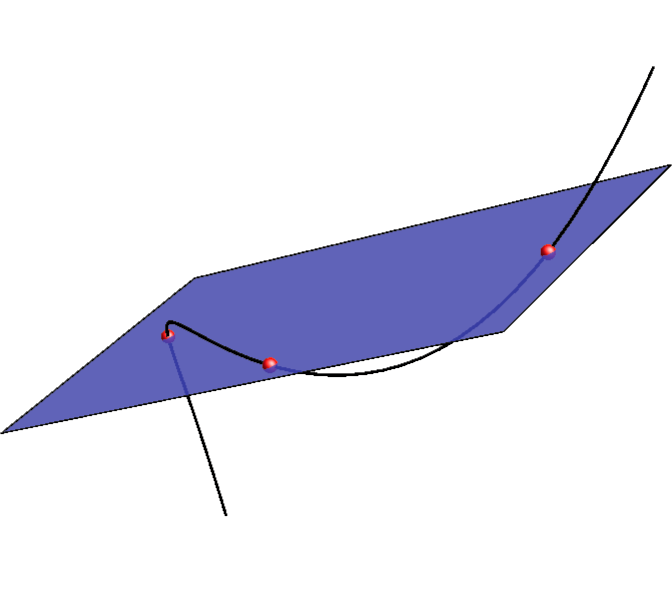Space curve of the 3rd order.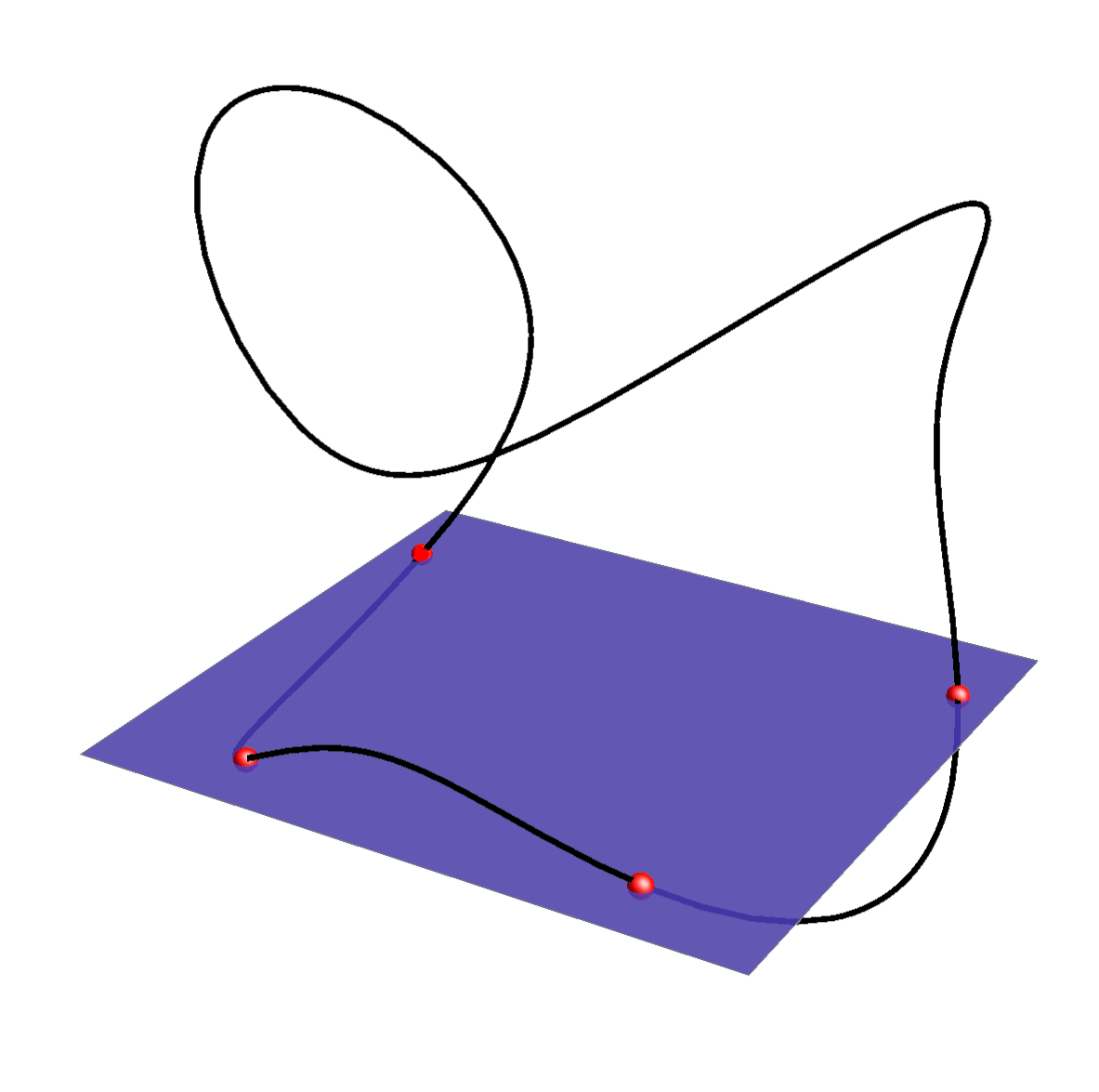One-branch space curve of the 4th order.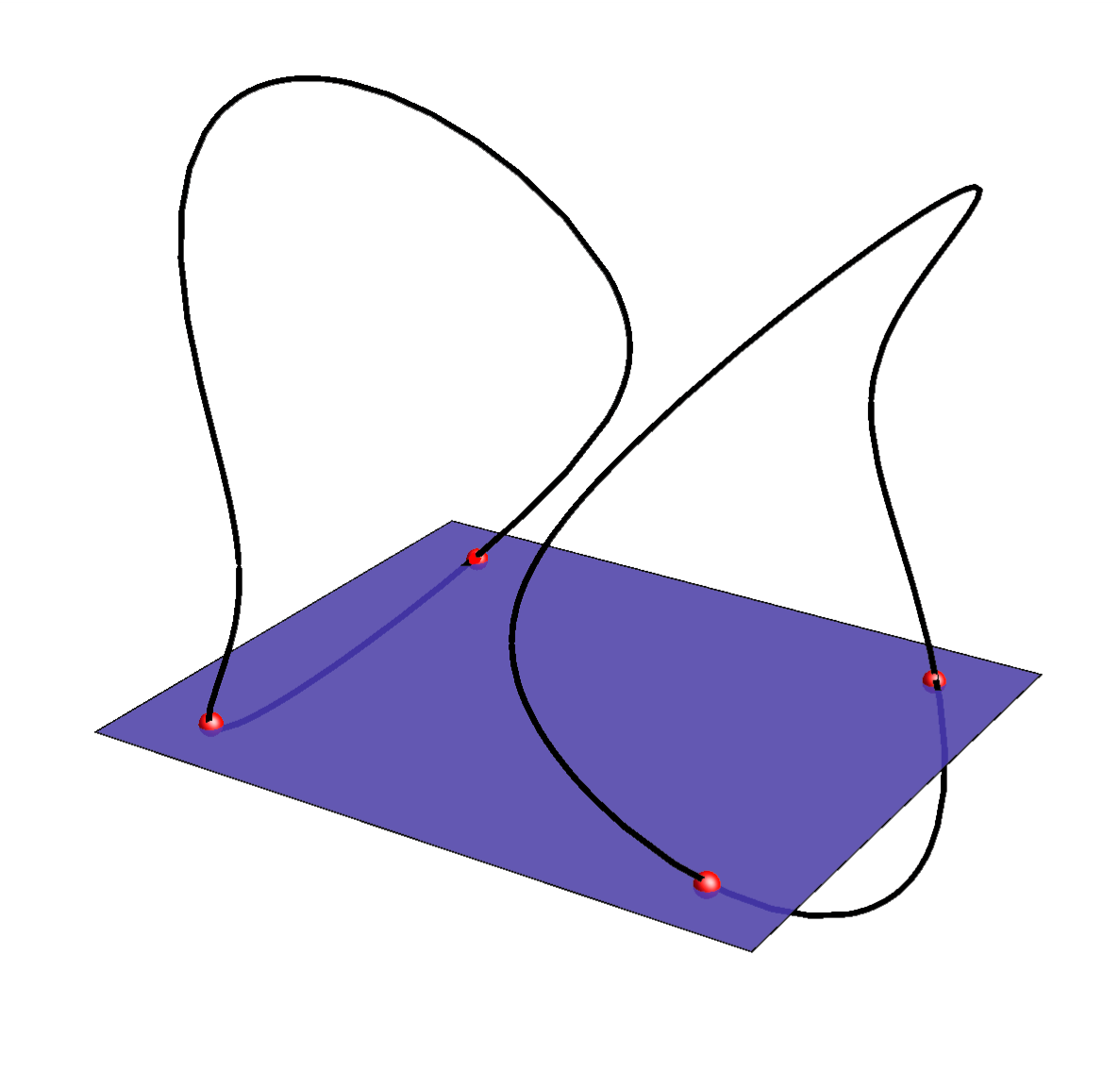Two-branch space curve of the 4th order.

Algebraic space curve can have multiple points (double points, triple points, ...). The number of double points is bounded by the order of the curve and if the number of double points exceeds this bound, the curve is decomposed into curves of lower order.

Maximal number of double points of proper space curve of the $$n$$th order equals:

• $$\frac{(n-2)^2}{4}$$, for $$n$$ even,

• $$\frac{(n-1)(n-3)}{4}$$, for $$n$$ odd.

If the number of double points is greater than this bound, then the curve of the $$n-$$th order is decomposed into curves of lower order so that the sum of their orders equals n.

#### Double points on a space curve

What is the condition for a point on the intersection curve of two surfaces to be a double point?
 Let $$k$$ be the intersection curve of the surfaces $$\Phi$$ and $$\Psi$$ and let the point $$T\in k$$ be a regular point on $$\Phi$$ and $$\Psi$$. The point $$T$$ is a double point of the curve $$k$$ if and only if the surfaces $$\Phi$$ and $$\Psi$$ have a common tangent plane in that point.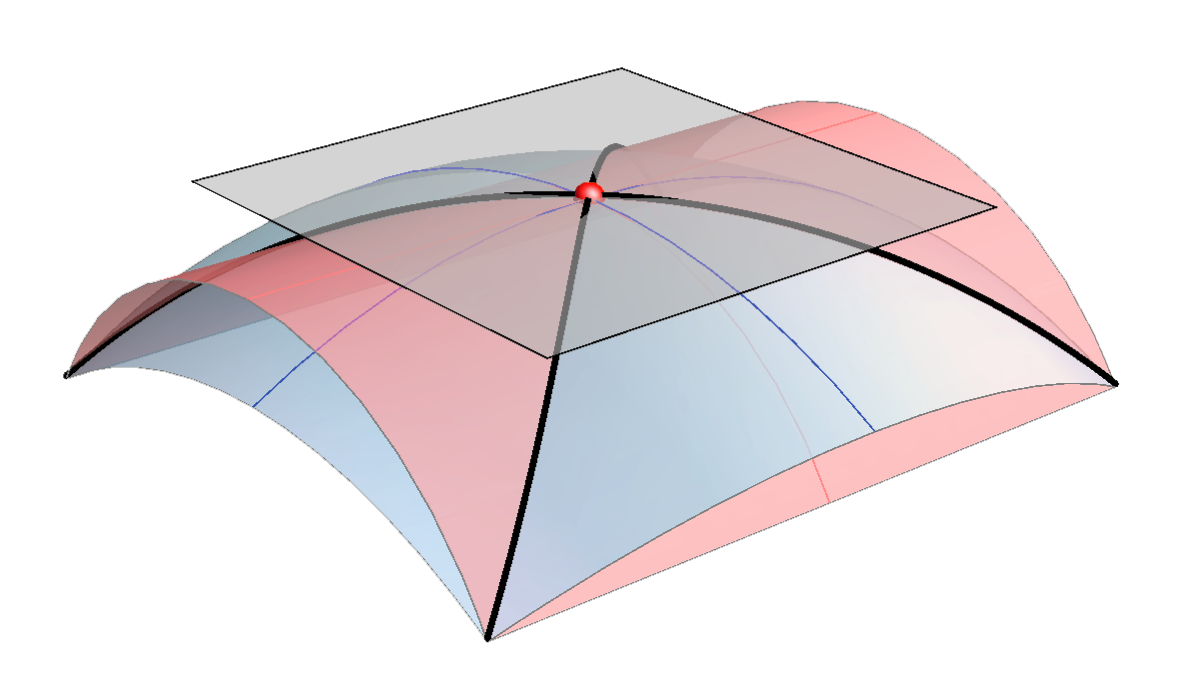Beside that, a point which is a double point of a surface is also a double point of any intersection curve that passes through that point.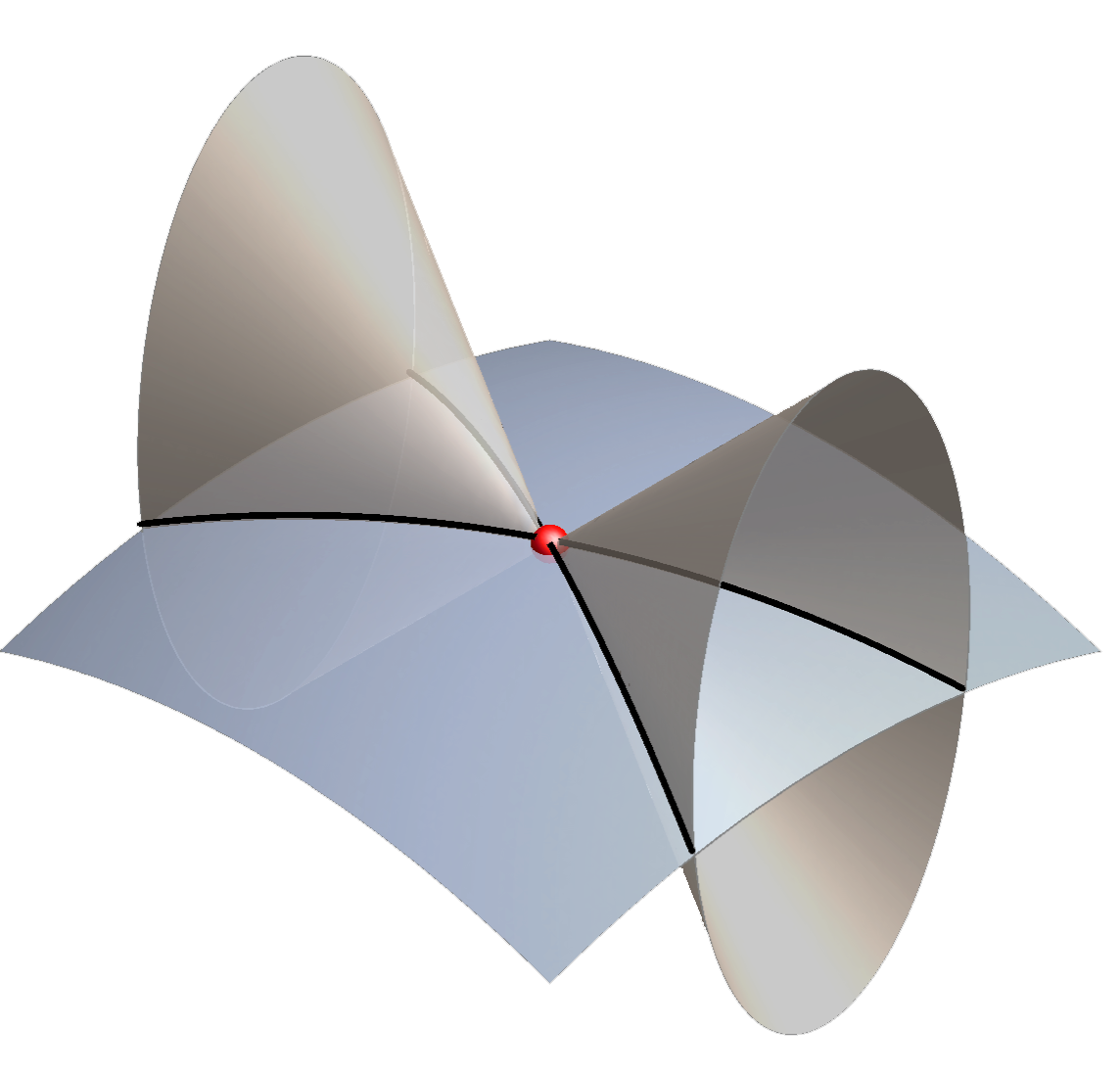#### Tangent line of an intersection curve

The tangent plane of a surface contains the tangent lines of all curves that lie on that surface, so we conclude the following:

• Tangent line of an intersection curve at a regular point is the
intersection line of the tangent planes of the surfaces in that point.

• right-click $$\rightarrow$$ PLAY

Space curve of the 4th order obtained as the intersection curve of cones, cylinders and spheres.

$$^*$$ One could make a mistake and conclude that there are no algebraic space curves of prime order. Such curves can be obtained as curves in the decomposition of intersection curves. We will give some examples for the curves of the 3rd order.

Created by Sonja Gorjanc, translated by Helena Halas and Iva Kodrnja - 3DGeomTeh - Developing project of the University of Zagreb